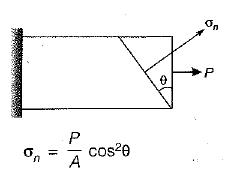Courses

# Test: Principal Stress & Strain - 1

## 10 Questions MCQ Test Topicwise Question Bank for Civil Engineering | Test: Principal Stress & Strain - 1

Description
This mock test of Test: Principal Stress & Strain - 1 for Civil Engineering (CE) helps you for every Civil Engineering (CE) entrance exam. This contains 10 Multiple Choice Questions for Civil Engineering (CE) Test: Principal Stress & Strain - 1 (mcq) to study with solutions a complete question bank. The solved questions answers in this Test: Principal Stress & Strain - 1 quiz give you a good mix of easy questions and tough questions. Civil Engineering (CE) students definitely take this Test: Principal Stress & Strain - 1 exercise for a better result in the exam. You can find other Test: Principal Stress & Strain - 1 extra questions, long questions & short questions for Civil Engineering (CE) on EduRev as well by searching above.
QUESTION: 1

### The state of stress at a point in a loaded member is shown in figure. The magnitude of maximum shear stress is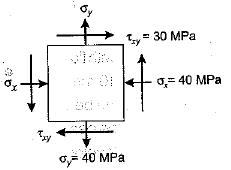Solution: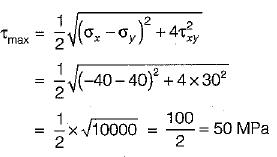QUESTION: 2

### At a point in a strained body carrying two unequal unlike principal stresses p1 and p2 (p1 > p2), the maximum shear stress is given by

Solution:

The maximum shear stress is given by,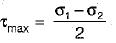Now, σ1 = +p1
σ2 = -p2
∴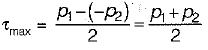QUESTION: 3

### The radius of Mohr’s circle of stress of a strained element is 20 N/mm2 and minor principal tensile stress is 10 N/mm2; The major principal stress is

Solution:

Minor principal stress = C - r = 10
Centre of circle (C) = 10 + 20 =30 N/mm2
Major principal stress = C + r = 30 + 20 = 50 N/mm2

QUESTION: 4

The principal stresses σ1 , σ2  and σ3 at a point respectively are 80 MPa, 30 MPa and -40 MPa. The maximum shear stress is

Solution: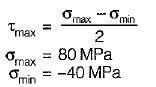∴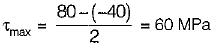QUESTION: 5

Plane stress at a point in a body is defined by principal stress 3σ and σ. The ratio of the normal stress to the maximum shear stress on the plane of maximum shear stress is

Solution: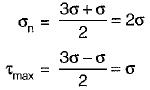∴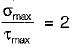QUESTION: 6

A shaft subjected to torsion experiences a pure shear stress τ on the surface. The maximum principal stress on the surface which is at 45° to the axis will have a value

Solution: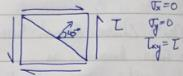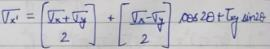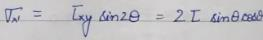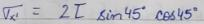QUESTION: 7

Principal strains at a point are 100 x 10-6 and -200 x 10-6. What is the maximum shear strain at the point?

Solution: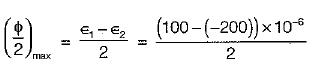= 150 x 10-6
∴  φmax = 300 x 10-6

QUESTION: 8

In a plane stress problem there are normal tensile stresses σx and σy accompanied by shear stress τxy at a point along orthogonal Cartesian coordinates x and y respectively. If it is observed that the minimum principal stress on a certain plane is zero the

Solution:

Principal stresses,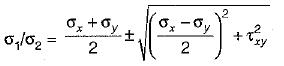Since minimum principal stress is zero, therefore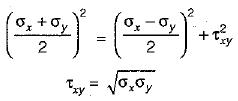QUESTION: 9

A point in a strained body is subjected to a tensile stress of 100 MPa on one plane and a tensile stress of 50 MPa on a plane at right angle to it. If these planes are carrying shear stresses of 50 MPa, then the principal stresses are inclined to the larger normal stress at an angle of

Solution:

The angle of inclination of principal stress to the larger normal stress is given by,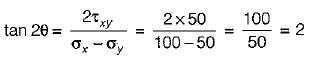⇒ 2θ = tan-1(2)
∴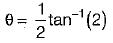QUESTION: 10

If the normal cross-section A of a member is subjected to a tensile force P, the resulting normal stress on an oblique plane inclined at angle θ to transverse plane will be

Solution: